Type
Quiz
Book Title
Fundamentals of Corporate Finance Standard Edition 9th Edition
ISBN 13
978-0073382395

### 978-0073382395 Chapter 13 Questions and Problems 1-17

April 3, 2019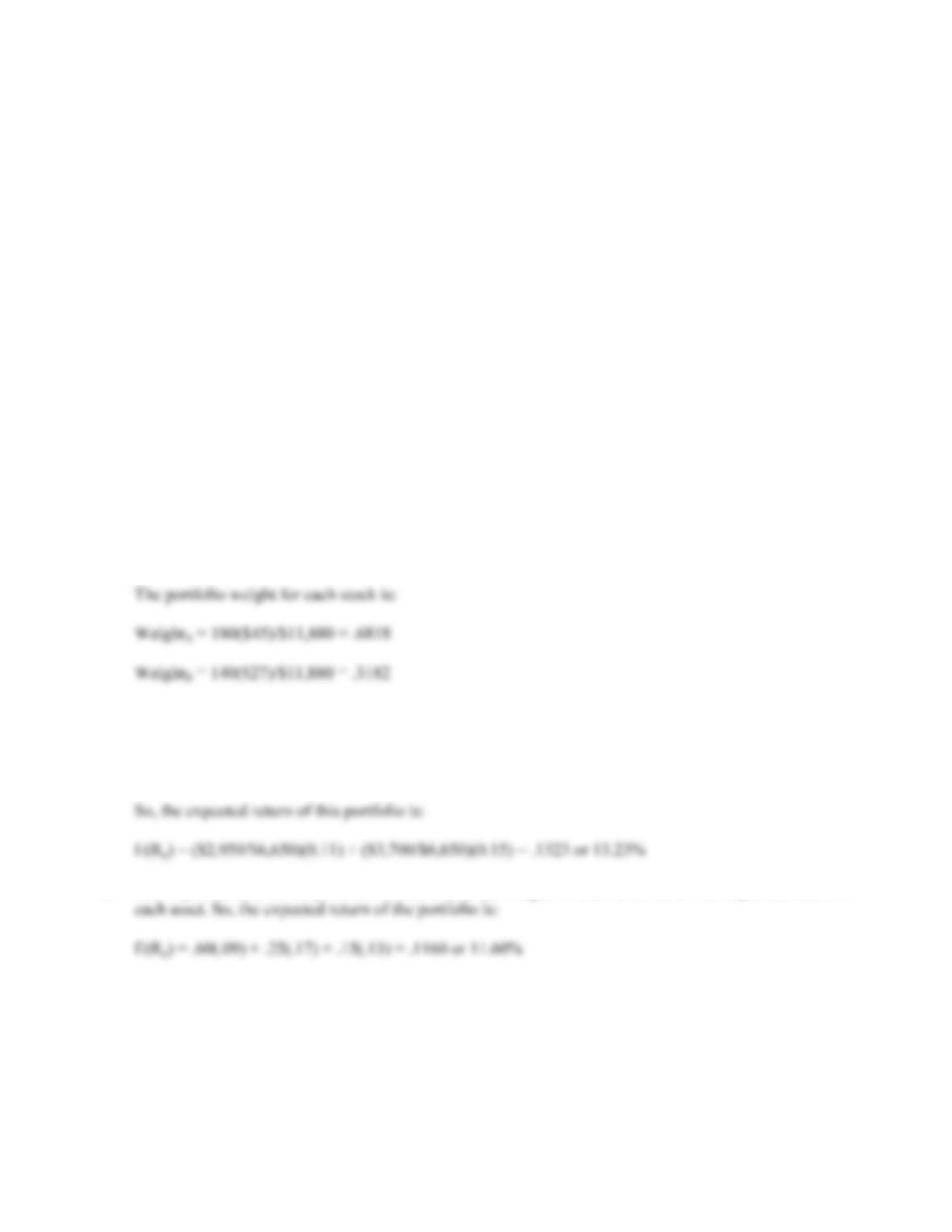CHAPTER 13 B-237
9. Such layoffs generally occur in the context of corporate restructurings. To the extent that the market
views a restructuring as value-creating, stock prices will rise. So, it’s not layoffs per se that are being
cheered on. Nonetheless, Wall Street does encourage corporations to takes actions to create value, even
if such actions involve layoffs.
10. Earnings contain information about recent sales and costs. This information is useful for projecting
future growth rates and cash flows. Thus, unexpectedly low earnings often lead market participants to
reduce estimates of future growth rates and cash flows; price drops are the result. The reverse is
often true for unexpectedly high earnings.
Solutions to Questions and Problems
NOTE: All end of chapter problems were solved using a spreadsheet. Many problems require multiple steps.
Due to space and readability constraints, when these intermediate steps are included in this solutions
manual, rounding may appear to have occurred. However, the final answer for each problem is found
without rounding during any step in the problem.
Basic
1. The portfolio weight of an asset is total investment in that asset divided by the total portfolio value.
First, we will find the portfolio value, which is:
Total value = 180(\$45) + 140(\$27) = \$11,880
2. The expected return of a portfolio is the sum of the weight of each asset times the expected return of
each asset. The total value of the portfolio is:
Total value = \$2,950 + 3,700 = \$6,650
3. The expected return of a portfolio is the sum of the weight of each asset times the expected return of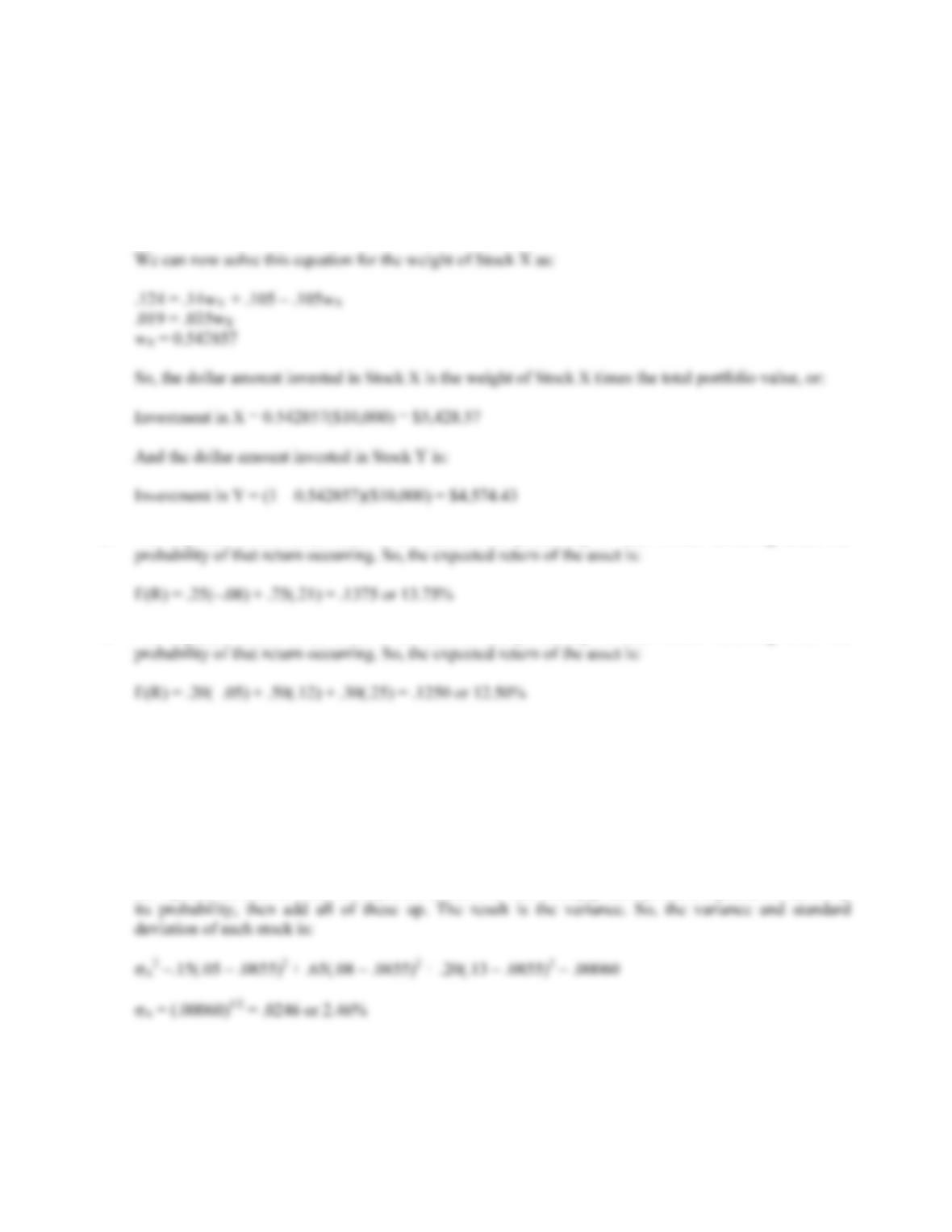B-238 SOLUTIONS
4. Here we are given the expected return of the portfolio and the expected return of each asset in the
portfolio, and are asked to find the weight of each asset. We can use the equation for the expected return
of a portfolio to solve this problem. Since the total weight of a portfolio must equal 1 (100%), the
weight of Stock Y must be one minus the weight of Stock X. Mathematically speaking, this means:
E(Rp) = .124 = .14wX + .105(1 – wX)
5. The expected return of an asset is the sum of the probability of each return occurring times the
6. The expected return of an asset is the sum of the probability of each return occurring times the
7. The expected return of an asset is the sum of the probability of each return occurring times the
probability of that return occurring. So, the expected return of each stock asset is:
E(RA) = .15(.05) + .65(.08) + .20(.13) = .0855 or 8.55%
E(RB) = .15(–.17) + .65(.12) + .20(.29) = .1105 or 11.05%
To calculate the standard deviation, we first need to calculate the variance. To find the variance, we find
the squared deviations from the expected return. We then multiply each possible squared deviation by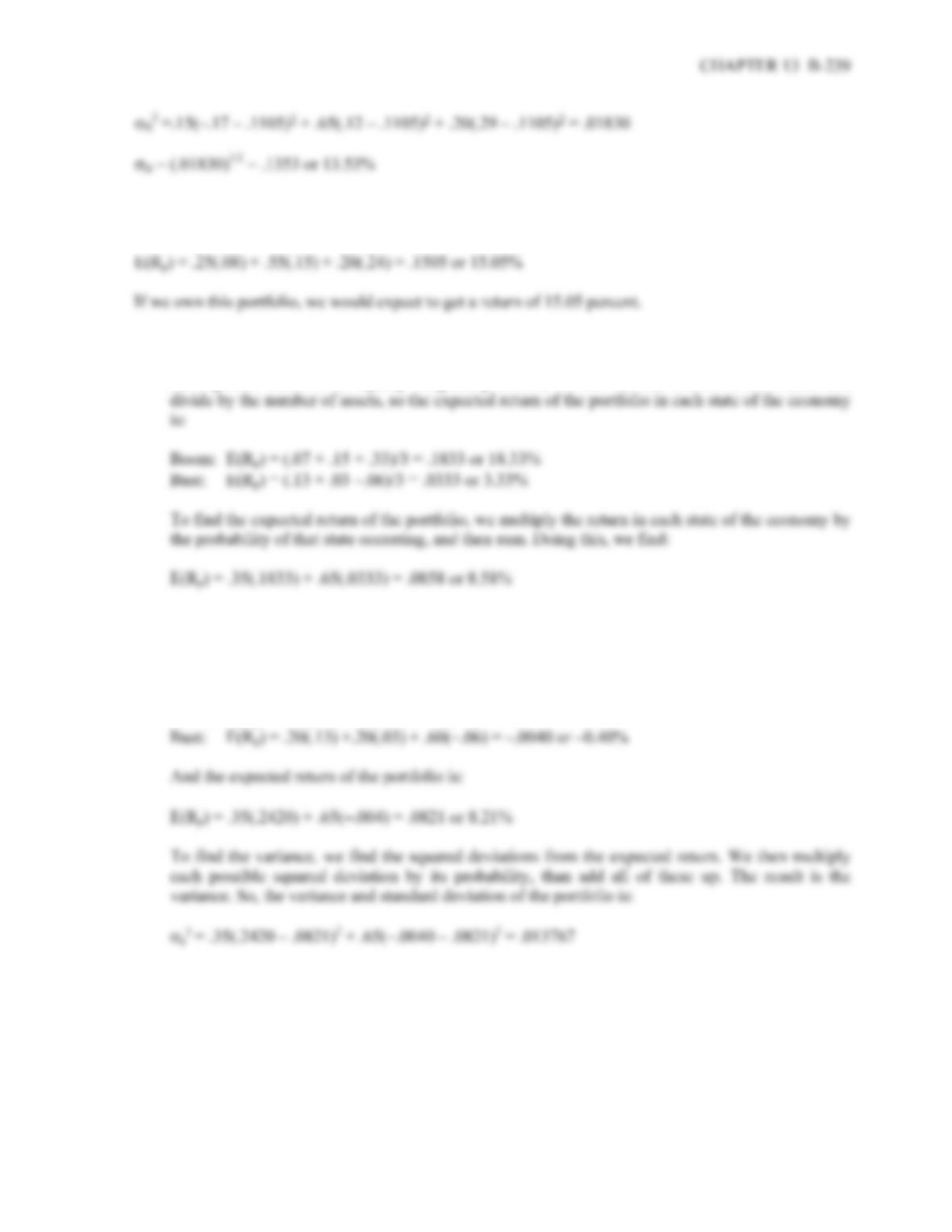8. The expected return of a portfolio is the sum of the weight of each asset times the expected return of
each asset. So, the expected return of the portfolio is:
9. a. To find the expected return of the portfolio, we need to find the return of the portfolio in each state
of the economy. This portfolio is a special case since all three assets have the same weight. To find
the expected return in an equally weighted portfolio, we can sum the returns of each asset and
b. This portfolio does not have an equal weight in each asset. We still need to find the return of the
portfolio in each state of the economy. To do this, we will multiply the return of each asset by its
portfolio weight and then sum the products to get the portfolio return in each state of the economy.
Doing so, we get:
Boom: E(Rp) = .20(.07) +.20(.15) + .60(.33) =.2420 or 24.20%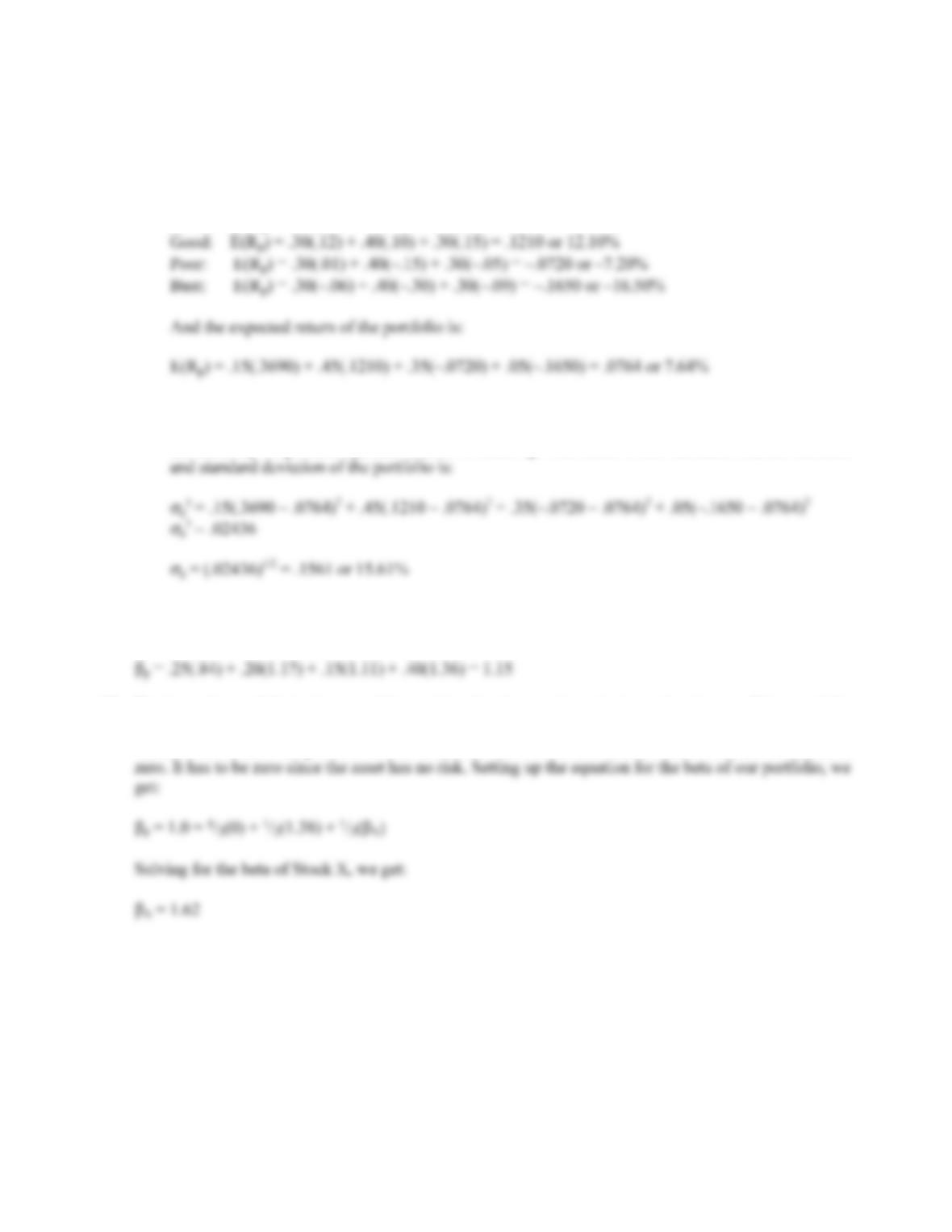B-240 SOLUTIONS
10. a. This portfolio does not have an equal weight in each asset. We first need to find the return of the
portfolio in each state of the economy. To do this, we will multiply the return of each asset by its
portfolio weight and then sum the products to get the portfolio return in each state of the economy.
Doing so, we get:
Boom: E(Rp) = .30(.3) + .40(.45) + .30(.33) = .3690 or 36.90%
b. To calculate the standard deviation, we first need to calculate the variance. To find the variance,
we find the squared deviations from the expected return. We then multiply each possible squared
deviation by its probability, than add all of these up. The result is the variance. So, the variance
11. The beta of a portfolio is the sum of the weight of each asset times the beta of each asset. So, the beta of
the portfolio is:
12. The beta of a portfolio is the sum of the weight of each asset times the beta of each asset. If the portfolio
is as risky as the market it must have the same beta as the market. Since the beta of the market is one,
we know the beta of our portfolio is one. We also need to remember that the beta of the risk-free asset is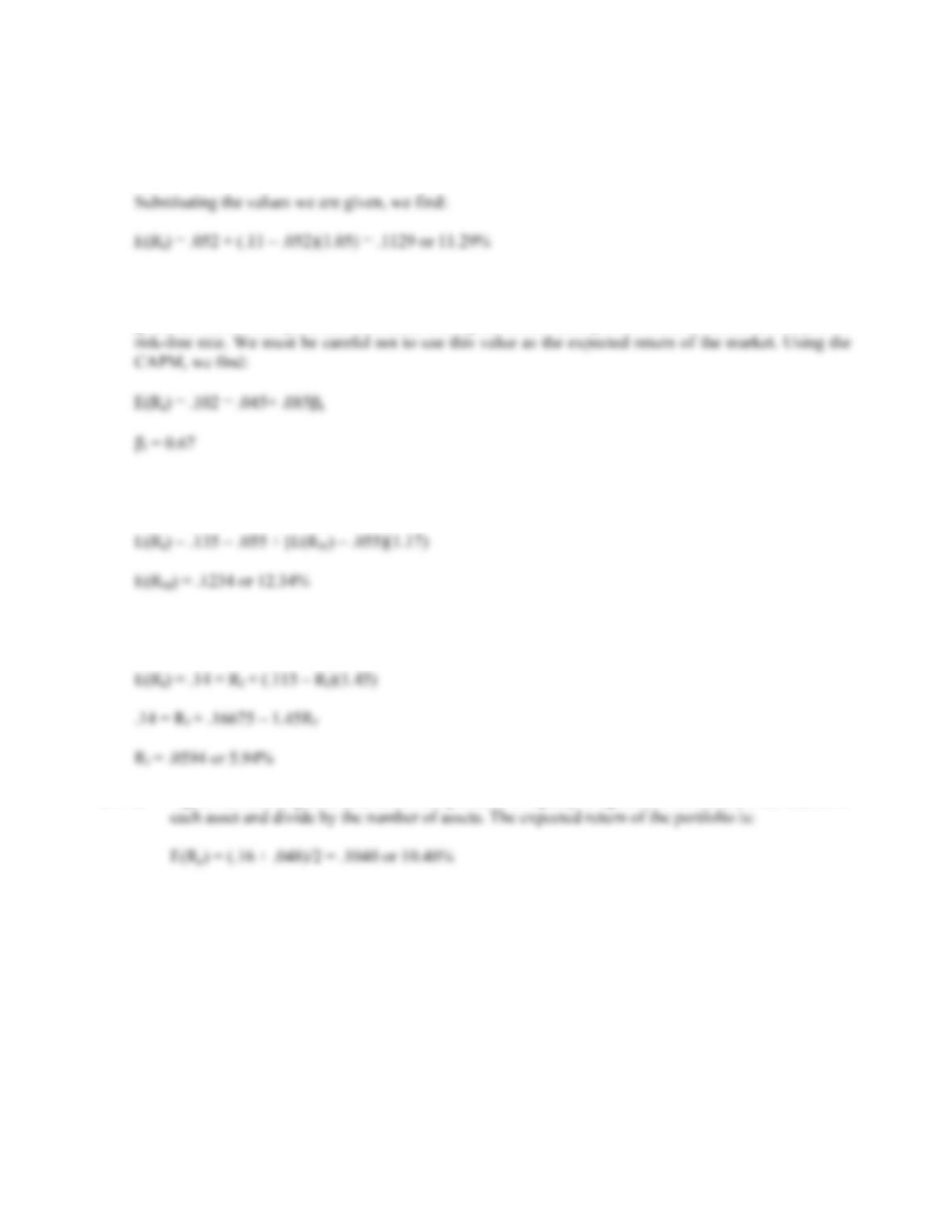CHAPTER 13 B-241
13. CAPM states the relationship between the risk of an asset and its expected return. CAPM is:
E(Ri) = Rf + [E(RM) – Rf] × i
14. We are given the values for the CAPM except for the of the stock. We need to substitute these values
into the CAPM, and solve for the of the stock. One important thing we need to realize is that we are
given the market risk premium. The market risk premium is the expected return of the market minus the
15. Here we need to find the expected return of the market using the CAPM. Substituting the values given,
and solving for the expected return of the market, we find:
16. Here we need to find the risk-free rate using the CAPM. Substituting the values given, and solving for
the risk-free rate, we find:
17. a. Again we have a special case where the portfolio is equally weighted, so we can sum the returns of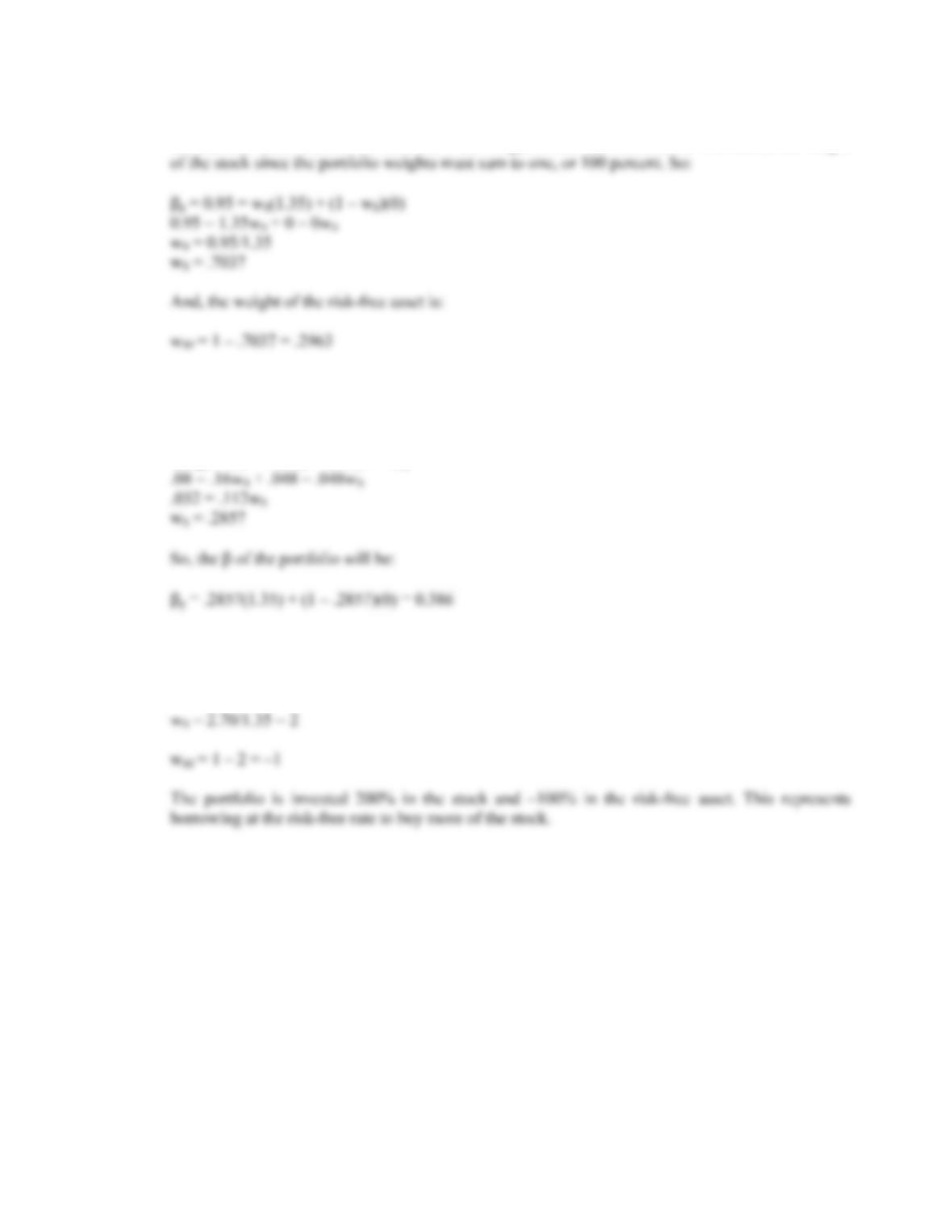B-242 SOLUTIONS
b. We need to find the portfolio weights that result in a portfolio with a of 0.95. We know the of
the risk-free asset is zero. We also know the weight of the risk-free asset is one minus the weight
c. We need to find the portfolio weights that result in a portfolio with an expected return of 8 percent.
We also know the weight of the risk-free asset is one minus the weight of the stock since the
portfolio weights must sum to one, or 100 percent. So:
E(Rp) = .08 = .16wS + .048(1 – wS)
d. Solving for the of the portfolio as we did in part a, we find:
p = 2.70 = wS(1.35) + (1 – wS)(0)
w
18. First, we need to find the of the portfolio. The of the risk-free asset is zero, and the weight of the
risk-free asset is one minus the weight of the stock, the of the portfolio is:
ß
p = wW(1.25) + (1 – wW)(0) = 1.25wW
So, to find the of the portfolio for any weight of the stock, we simply multiply the weight of the stock
times its .